# GpGp Analysis of Jason-3 Wind Data

#### 2018-02-18

The windspeed data in this vignette comes from the Jason-3 satellite and has been lightly preprocessed to remove data points near cloud and ice obstructions and to average over 10 second intervals. A more complete description of the data and the analysis can be found in Guinness (2018, Technometrics).

We first load the GpGp library, read in the data, and asisgn some variables.

library("GpGp")
data(jason3)
#>   windspeed      lon       lat     time
#> 1    15.846 56.11859 -59.77674 360.1140
#> 2    15.838 56.93162 -59.45146 370.3011
#> 3    14.795 57.72831 -59.12069 380.4882
#> 4    15.273 58.50881 -58.78459 390.6753
#> 5    16.031 59.27350 -58.44332 400.8624
#> 6    15.113 60.02259 -58.09707 411.0495
lat       <- jason3$lat lon <- jason3$lon
windspeed <- jason3$windspeed time <- jason3$time
n         <- length(windspeed)

## Visualizing the Data

Each windspeed value (m/s) comes with a longitude, latitude, and time value. We can visualize the spatial-temporal structure of the data by making quilt plots (from the fields package) of the first six hours of data, the first 24 hours of data, and from the entire dataset. We can see how the satellite improves its spatial coverage over longer time periods. The time period of this dataset is about 6 days, during which wind speeds can change substantially.

# plot of data from first 6 hours
par(mar=c(4,4,1,1))
inds <- time < 6*3600
fields::quilt.plot(lon[inds],lat[inds],windspeed[inds],
nx=400,ny=200,xlab="Lon",ylab="Lat",legend.lab = "windspeed (m/s)")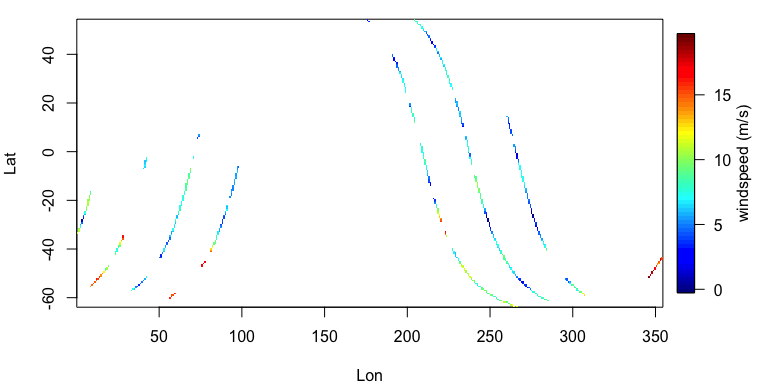# plot of data from first day
inds <- time < 24*3600
fields::quilt.plot(lon[inds],lat[inds],windspeed[inds],
nx=400,ny=200,xlab="Lon",ylab="Lat",legend.lab = "windspeed (m/s)")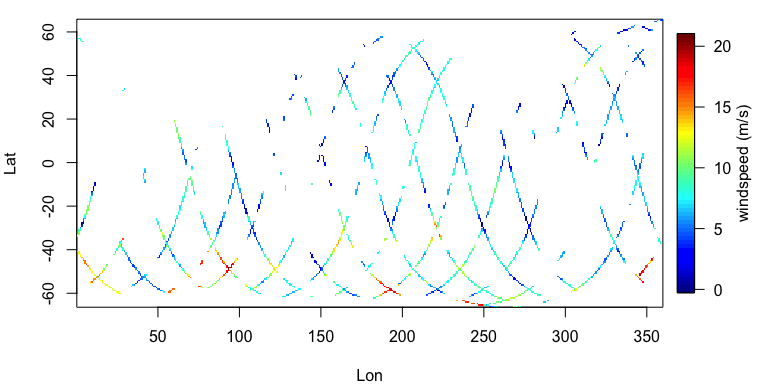# plot of all data
fields::quilt.plot(lon,lat,windspeed,
nx=400,ny=200,xlab="Lon",ylab="Lat",legend.lab = "windspeed (m/s)")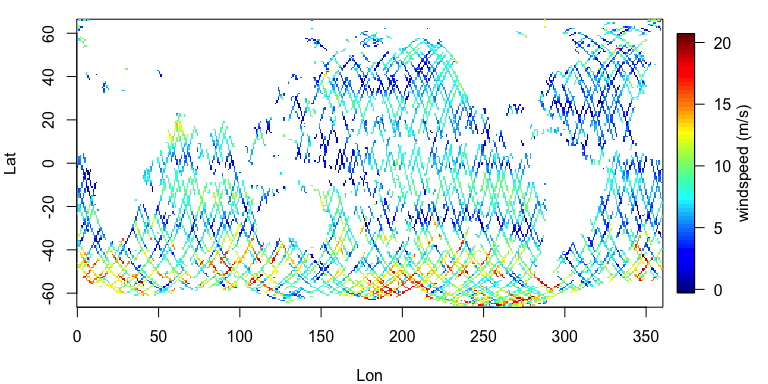## Preparing Variables for Fitting

The fit_model function requires a response vector, a matrix of locations, and optionally, a design matrix of predictors. If the design matrix is not specified, the function will assume a constant mean. For demonstration purposes here, we explicitly specify the design matrix as a matrix with a single column of ones.

# longitude latitude locations, space-time locations, and design matrix
locs <- cbind(lon,lat)
locstime <- cbind(locs,time)
X <- as.matrix( rep(1,length(windspeed)) )

## Model Fitting

We fit two models here, both of which use the Mat'ern covariance function. The first ignores the temporal dimension and treats the data as being collected simultaneously. This approach is sometimes appropriate for spatial-temporal data that do not change much over time. The second model includes the temporal component. We think the second model is more appropriate because windspeeds can change substantially over six days, and so our motivation for showing both models is to demonstrate the importance of considering the temporal component carefully.

The two models take several minutes to fit on my system.

# fit to a subset of the data (for faster CRAN checks)
# use 'inds <- 1:n' to fit to full dataset
inds <- round( seq(1,n,length.out = 1500) )
fit_space     <- fit_model(windspeed[inds], locs[inds,], X[inds,], "matern_sphere")
#> Using 'matern_sphere'. Assuming that argument 'locs' is (longitude,latitude)
#> Reordering...Done
#> Finding nearest neighbors...Done
#> Refining estimates...Done          45.139 45.578 0.767
#> Refining estimates...Done          0.081 45.578 1.482
#> Refining estimates...Done          0.078 45.578 1.489
fit_spacetime <- fit_model(windspeed[inds], locstime[inds,], X[inds,], "matern_sphere_time")
#> Using 'matern_sphere_time'. Assuming that argument 'locs' is (longitude,latitude,time)
#> Reordering...Done
#> Finding nearest neighbors...Done
#> Refining estimates...Done          0.138 2019683.339 0.692 0.135
#> Refining estimates...Done          0.222 214530.651 0.349 0.001
#> Refining estimates...Done          0.198 192512.949 0.396 0
fit_space
#> $loglik #>  -3715.983 #> #>$covparms
#>   5.29753675  0.07773544 45.57782417  1.48859182
#>
#> $beta #> [,1] #> [1,] 7.696671 #> #>$betacovmat
#>          [,1]
#> [1,] 1.336759
fit_spacetime
#> $loglik #>  -3549.221 #> #>$covparms
#>  1.069068e+01 1.975954e-01 1.925129e+05 3.959020e-01 1.240163e-04
#>
#> $beta #> [,1] #> [1,] 7.051337 #> #>$betacovmat
#>           [,1]
#> [1,] 0.1323973

The first thing to note is that the space-time model gives a much higher loglikelihood. The fitted model objects contain an element covparms, which is a vector with the estimated variance, spatial range, temporal range (space-time model only), smoothness, and nugget parameters. The second covariance parameter in both models is the spatial range parameter, in units of radians (i.e. Earth radius = 1). The space-time model has a larger spatial range. This result stems from the fact that when the satellite crosses back over its own path, the measurements from the two paths may not coincide, even though they are close spatially.

The space-time model knows that these measurements were taken at different time points, and so does not take the non-coinciding measurements as evidence that the spatial correlation is weak. The space-only model does not know that the measurements were taken at different times, and so does take this as evidence that the spatial correlation is weak. For the same reason, the space-only model has a larger nugget parameter.

# Predictions and Conditional Simulations

The package also has functions for making predictions at unobserved spatial or spatial-temporal locations. For the wind data, we predict the entire wind field at a time point in the middle of the domain. The package is also capable of performing a conditional simulation, which can be thought of as attempt to reconstruct a realistic-looking wind field that is consistent with the observed data. Prediction maps tend to be too smooth, whereas conditional simulations add the noise back in.’’ The noise should not be taken literally as the exact wind field, but instead as one plausible wind field, given the information in the data. Ensembles of several conditional simulations can serve as a useful tool for quantifying uncertainties in predictins, especially when the prediction of interest is a non-linear function of the unobserved data; for example, the number of pixels exceeding a particular value.

# prediction locations and design matrix
mediantime <- median(time)
latgrid <- seq( min(lat), max(lat), length.out = 60 )
longrid <- seq( 0, 360, length.out = 121)[1:120] # so no locations repeated
locs_pred <- as.matrix( expand.grid(longrid,latgrid) )
n_pred <- nrow(locs_pred)
locstime_pred <- cbind( locs_pred, rep(mediantime, n_pred) )
X_pred <- as.matrix( rep(1,n_pred) )
# predictions
pred <- predictions(fit_spacetime$covparms, "matern_sphere_time", windspeed, locstime, locstime_pred, X, X_pred, fit_spacetime$beta, m = 40)
# plot predictions
par(mar=c(4,4,1,1))
pred_array <- array( pred, c(length(longrid),length(latgrid)) )
fields::image.plot(longrid,latgrid,pred_array)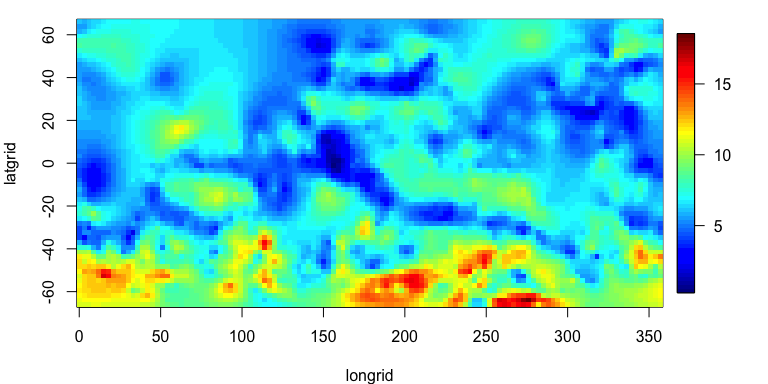# conditional simulations
sim <- cond_sim(fit_spacetime$covparms, "matern_sphere_time", windspeed, locstime, locstime_pred, X, X_pred, fit_spacetime$beta, m = 40)
# plot conditional simulations
sim_array <- array( sim, c(length(longrid),length(latgrid)) )
fields::image.plot(longrid,latgrid,sim_array)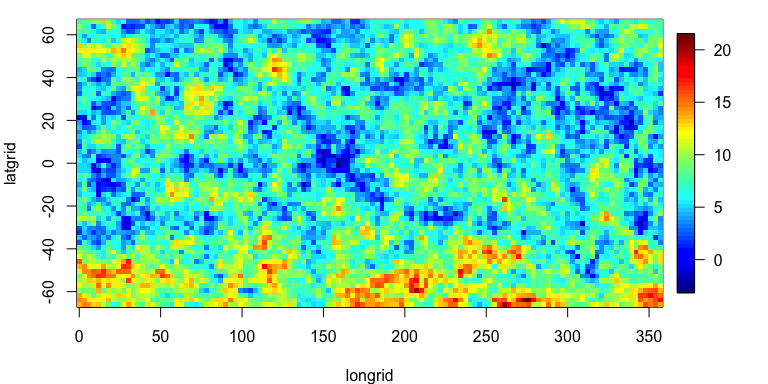The file vignette_windspeed_code.R in the vignettes directory of the source package (also available on github), contains the above code, as well as a more detailed description of the behind-the-scenes operations that fit_model hides from the user.